# Thermodynamics Class 11 Chemistry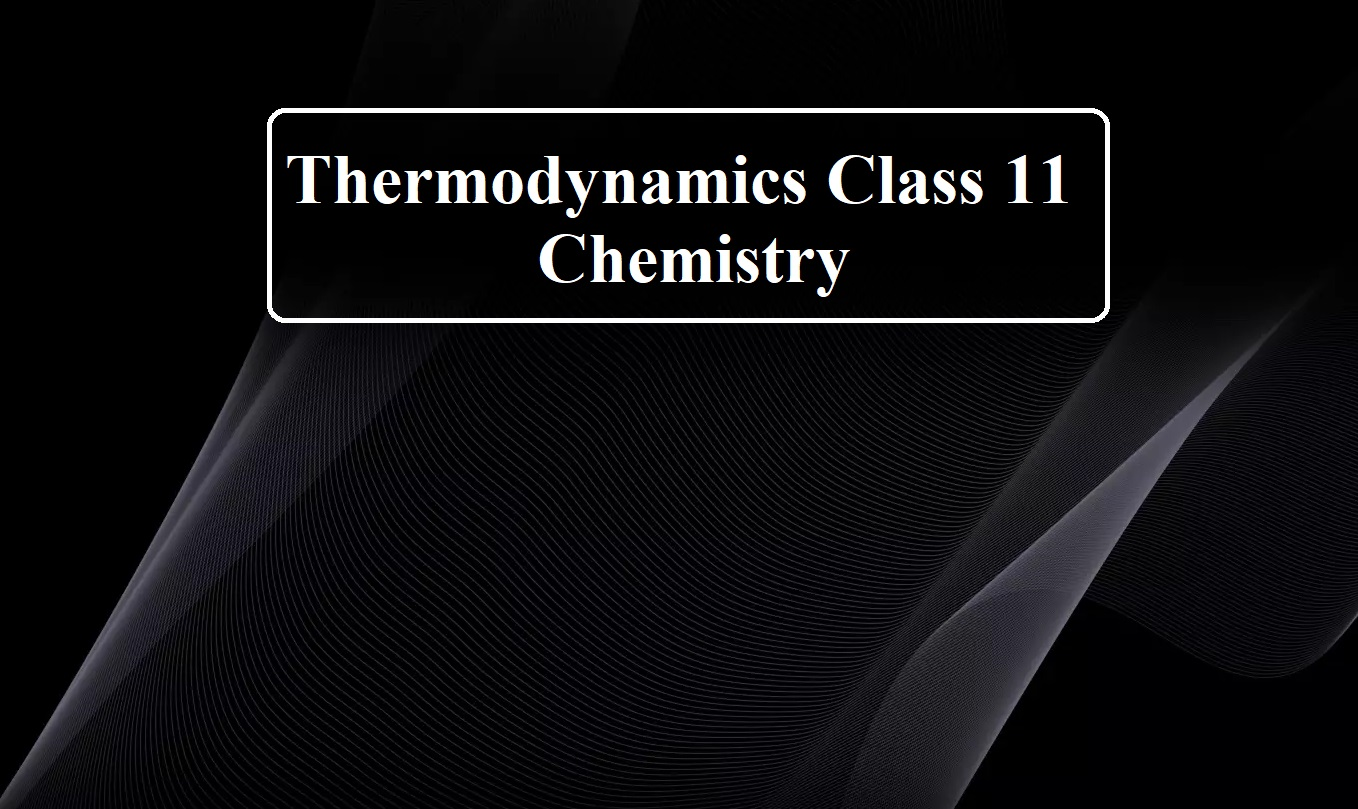# Thermodynamics Class 11 Chemistry

Thermodynamics Class 11 Chemistry

Thermodynamics (Greek word thermo means heat and dynamics means motion) is the branch of science which deals with the study of different forms of energy and the quantitative relationships between them. The study of thermodynamics is purely based upon three generalizations these are first, the second, and third law of thermodynamics.

Some important definitions involved:
System: A system is the part of the universe that is under observation or investigation.
Surroundings: The part of the universe except the system is called surroundings.
The system and surroundings are separated by a boundary that may be real or imaginary.
System + surroundings → Universe

1. Types of the system: Depending on the ability to exchange energy and matter with the surroundings, systems are classified into three types:
(i) Open system: This type of system can exchange matter as well as energy with its surroundings. The boundary is neither sealed nor insulated. Sodium reacting with water in an open beaker is an example of an open system.

(ii) Closed system: This type of system can exchange energy in the form of heat, work or radiations but not matter with its surroundings. The boundary between the system and surroundings is sealed but not insulated. For example, liquid in contact with vapour in a sealed tube and pressure cooker.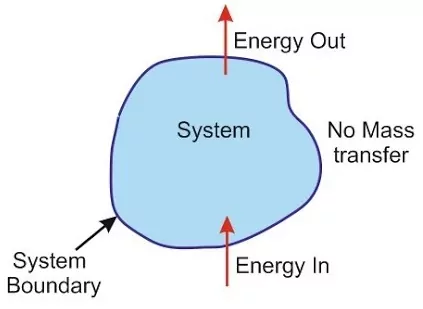(iii) Isolated system: This type of system has no interaction with its surroundings. The boundary is sealed and insulated. Neither matter nor energy can be exchanged with surroundings. A substance contained in an ideal thermos flask is an example of an isolated system.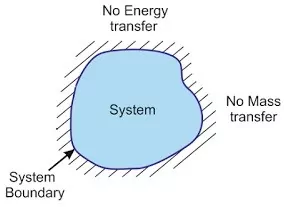Thermodynamics Class 11 Chemistry

Depending on the number of particles, systems are of two types:
(i) Microscopic system: It is a system containing a few number of particles.
(ii) Macroscopic system: it is a system that contains a large number of particles.
The properties of a macroscopic system are called macroscopic properties. Thermodynamics deals with matter in terms of bulk (a large number of chemical species) behaviour. The properties of the system which arise from the bulk behaviour of matter are called macroscopic properties. The important macroscopic properties are Temperature (T), Pressure (P), Volume (V), length (l), breadth (b), height (h), internal energy (U), enthalpy (H), entropy (S), Gibb’s energy (G) etc.

Thermodynamics Class 11 Chemistry

The macroscopic properties can be subdivided into two types:
(i) Intensive Properties: The properties which do not depend upon the quantity of matter present in the system or size of the system are called intensive properties. For example Temperature (T), Pressure (P), Volume (V), density, refractive index, molar heat capacity, viscosity, surface tension etc.
(ii) Extensive Properties: These are properties that depend on the amount of matter present in the system. Or, these are the properties that change when a system is divided. E.g.: Volume (v), length (l), breadth (b), height (h), internal energy (U), enthalpy (H), entropy (S), Gibb’s energy (G), heat capacity etc.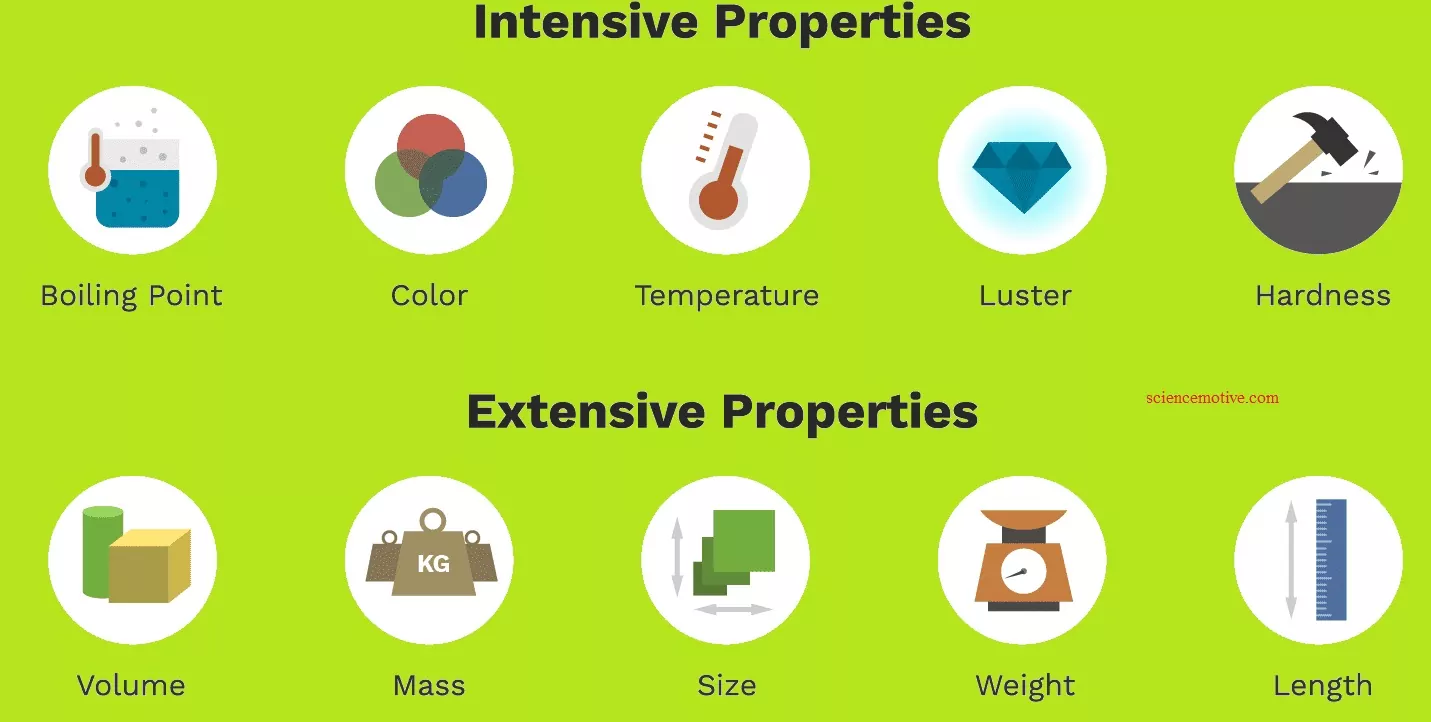(2) Homogeneous system: A system is said to be homogeneous when it is completely uniform throughout. A homogeneous system is made of one phase only. Examples: a pure single solid, liquid or gas, a mixture of gases and a true solution.
(3) Heterogeneous system: A system is said to be heterogeneous when it is not uniform throughout, i.e., it consists of two or more phases. Examples: ice in contact with water, two or more immiscible liquids, insoluble solid in contact with a liquid, a liquid in contact with vapour, etc.

State Function and Path Function:
Macroscopic properties which determine the state of a system are referred to as state variables or state functions or thermodynamic parameters.
Such thermodynamic functions which depend only on the initial and final states of the system and not on the path followed are called State Functions. For example, Temperature, Pressure, volume, Internal Energy, Enthalpy, Gibb’s energy etc.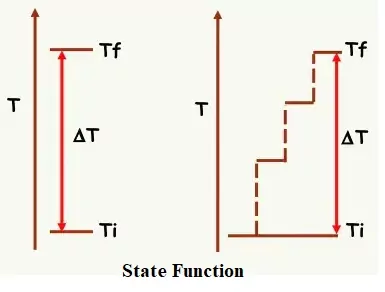The properties that depend on the path followed called Path Function. For example, Heat, Work etc.

Thermodynamic equilibrium: “A system is said to have attained a state of thermodynamic equilibrium when it shows no further tendency to change its property with time”.
The criterion for thermodynamic equilibrium requires that the following three types of equilibrium exist simultaneously in a system,
(i) Chemical Equilibrium: A system in which the composition of the system remains fixed and definite.
(ii) Mechanical Equilibrium: No chemical work is done between different parts of the system or between the system and surrounding. It can be achieved by keeping pressure constant.
(iii) Thermal Equilibrium: Temperature remains constant i.e. no flow of heat between system and surrounding.

Thermodynamic process: When the thermodynamic system changes from one state to another, the operation is called a process. The different types of thermodynamic process are:
i). Isothermal process: It is a process that takes place at constant temperature. For this process ΔT = 0 but Δq ≠ 0
ii). Isobaric process: It is a process that takes place at constant Pressure. For such a process ΔP = 0
iii). Isochoric process: It is a process that takes place at constant volume. For such a process ΔV = 0
iv). Adiabatic process: It is a process that takes place at constant heat energy. Here no heat enters into or leaves from the system. For such a process Δq= 0 but ΔT ≠ 0
v). Cyclic process: It is a process that takes place in a cyclic manner. Here the system undergoes a series of changes and finally returns to its initial state. For such a process ΔU=0 and ΔH = 0
vi). Reversible process: Every process is associated with two types of forces – driving force and opposing force. Driving force favours the process while opposing force opposes it. If the driving and opposing forces are differed by an infinitesimally small quantity the process takes place in both directions. Such a process is called reversible process.
vii). Irreversible process: When the process occurs from initial to final state in single step in finite time and cannot be reversed, it is termed an irreversible process. Amount of entropy increases in irreversible process. Irreversible processes are spontaneous in nature. All natural processes are irreversible in nature.

Thermodynamics Class 11 Chemistry